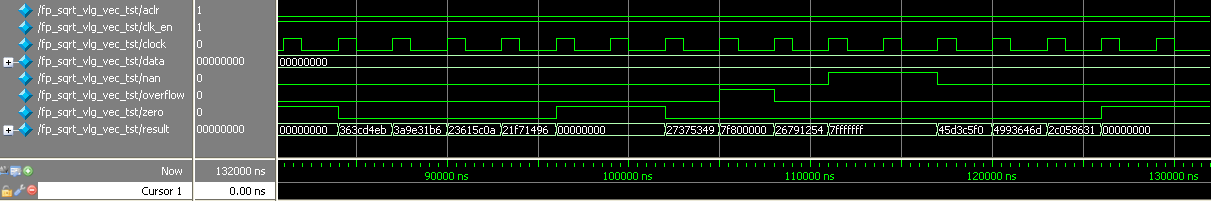ID 683750
Date 9/13/2021
Public

## 6.5.1. ALTFP_SQRT Design Example: Understanding the Simulation Results

The simulation waveform in this design example is not shown in its entirety. Run the design example files in the ModelSim* - Intel® FPGA Edition software to see the complete simulation waveforms.

These figures show the expected simulation results in the ModelSim* - Intel® FPGA Edition software.

Figure 20. ALTFP_SQRT Simulation Waveform (Input Data)Figure 21. ALTFP_SQRT Simulation Waveform (Output Data)This design example implements a floating-point square root function for single-precision format numbers with all the exception output ports instantiated. The output ports include overflow, zero, and nan.

The output latency is 28 clock cycles. Every square root computation generates the output result 28 clock cycles later.

Table 35.  Summary of Input Values and Corresponding OutputsThis table lists the inputs and corresponding outputs obtained from the simulation in the waveforms.
Time Event
0 ns, start-up Output value: All values seen on the output port before the 28th clock cycle are merely due to the behavior of the system during start-up and should be disregarded.
2 000 ns data[] value: 2D0B 496Ah

The data input is a normal number.

84 000 ns Output value: 363C D4EBh

The square root computation of a normal input results in a normal output.

14 000 ns data[] value: 0000 0000h
96 000 ns Output value: 0000 0000h

Exception handling ports: zero asserts

The square root computation of zero results in a zero.

23 000 ns data[] value: 7F80 0000h

The input is infinity.

105 000 ns Output value: 7F80 0000h

Exception handling ports: overflow asserts